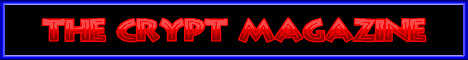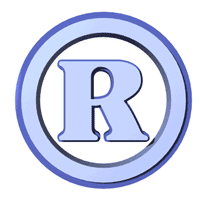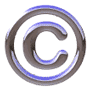Sponsored by BoysStuff.co.uk# Keyboard symbols and characters for WindowsEver wondered how you get the various symbols to appear in your text?  At some time or another we would all need to use these (eg. © ™ ¼ ½ ¾ )

The secret is Alt Key + Number = Symbol

Hold the ALT key and type the number next to the symbol you want on the CALCULATOR part of the keyboard, then release ALT key.  Symbol will appear.

Example: Hold ALT and type 0169 and you will get ©

Here are some other codes:

© = 0169

® = 0174

¤ = 15

¶ = 20

§ = 21

Ç = 128

ü = 129

é = 130

â = 131

ä = 132

à = 133

å = 134

ç = 135

ê = 136

ë = 137

è = 138

ï = 139

î = 140

ì = 141

Ä = 142

Å = 143

É = 144

æ = 145

Æ = 146

ô = 147

ö = 148

ò = 149

û = 150

ù = 151

ÿ = 152

Ö = 153

Ü = 154

¢ = 155

£ = 156

¥ = 157

ƒ = 159

á = 160

í = 161

ó = 162

ú = 163

ñ = 164

Ñ = 165

ª = 166

º = 167

¿ = 168

¬ = 170

½ = 171

¼ = 172

¡ = 173

« = 174

» = 175

¾ = 0190

ß = 225

µ = 230

± = 241

÷ = 246

• = 249

· = 250

² = 253

^ = 350

` = 352

~ = 382© RIYAN Productions